# Nonagon - Definition with Examples

The Complete K-5 Math Learning Program Built for Your Child

• 30 Million Kids

Loved by kids and parent worldwide

• 50,000 Schools

Trusted by teachers across schools

• Comprehensive Curriculum

Aligned to Common Core

What is Nonagon?

A nonagon is a polygon made up of 9 sides and 9 angles.

Nonagon = Nona + gon where Nona means nine and gon means sides.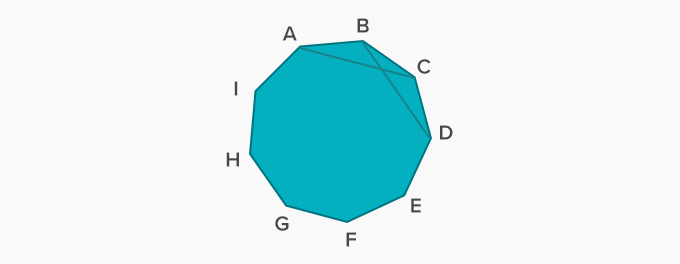The given nonagon ABCDEFGHI has sides AB, BC, CD, DE, EF, FG, GH, HI, and IA and angles ∠A, ∠B, ∠C, ∠D, ∠E, ∠F, ∠G, ∠H, and ∠I.

The blue line segments AC and BD are its diagonals.

There are total of 27 diagonals in a nonagon as shown below:Angles of a nonagon:

A nonagon consists of 9 angles. The sum of angles of a nonagon is 1260°.

A nonagon has 9 exterior angles. The sum of angles of the exterior angles of a nonagon is 360°.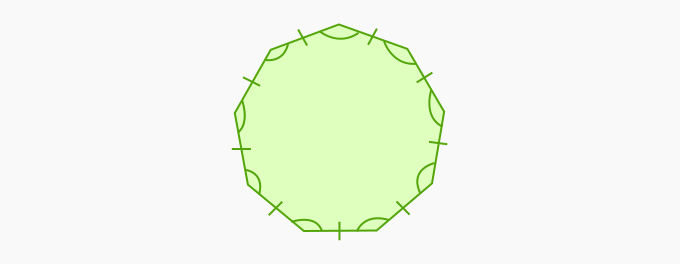Non-examples

The following shapes are not nonagons. Let us see why?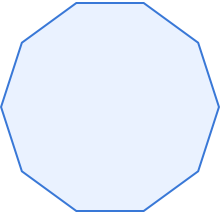There are more than 9 sides in this figure. So, it is not a nonagon.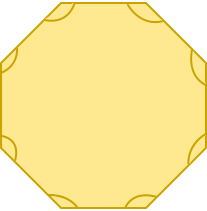There are 8 sides in this figure. So, it is not a nonagon.

Regular nonagon

A nonagon in which all sides are equal and all angles are equal is a regular nonagon.

Each angle of a regular nonagon is of 140°.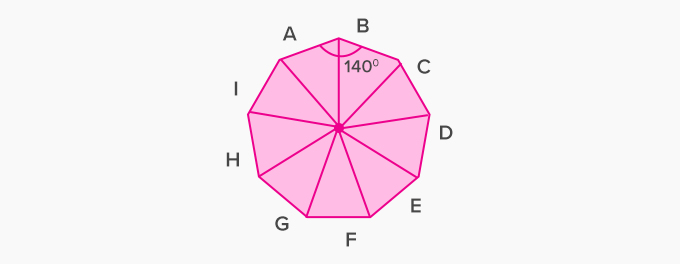Irregular nonagon

A nonagon in which all sides are not equal and all angles are not equal. The measure of its angles are different but total sum of all its interior angles is always 1260°.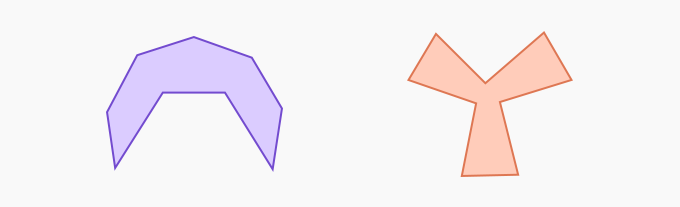Fun facts about nonagons A nonagon is also called enneagon, which is derived from a Greek word called enneagonon, which means nine corners. The first use of the word nonagon dates back to the early 17th century, and has a Latin prefix (nona) and a Greek suffix (gon), making it a polygon with nine sides and nine angles.

Won Numerous Awards & Honors